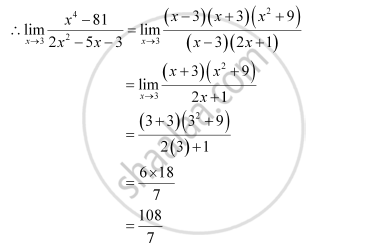CBSE (Science) Class 11CBSE
Share
Notifications

View all notifications
Books Shortlist
Your shortlist is empty

# Solution for Evaluate the Given Limit Lim_(X -> 3) (X^4 - 81)/(2x^2-5x - 3) - CBSE (Science) Class 11 - Mathematics

Login
Create free account

Forgot password?
ConceptIntuitive Idea of Derivatives

#### Question

Evaluate the Given limit lim_(x -> 3) (x^4 - 81)/(2x^2-5x - 3)

#### Solution

At x = 2, the value of the given rational function takes the form 0/0Is there an error in this question or solution?

#### APPEARS IN

NCERT Solution for Mathematics Textbook for Class 11 (2013 to Current)
Chapter 13: Limits and Derivatives
Q: 8 | Page no. 301
Solution Evaluate the Given Limit Lim_(X -> 3) (X^4 - 81)/(2x^2-5x - 3) Concept: Intuitive Idea of Derivatives.
S